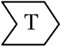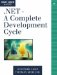# Unit TestsAs in the previous iterations, the implementation must be tested before the project can move on to the next iteration. The tests should verify that the new objects are working OK. In addition, the added Pen object must be tested.

Based on the unit tests that were developed in Chapter 6, we implement new test cases to verify the correct behavior of the units implemented in this iteration. The unit tests will look very much like the unit tests for the rectangle and circle components in Chapter 6 because the functionality is very similar.

This section shows the unit test implementation for the ellipse frame (see Listing 7.5). The EllipseFrameTest first writes to the console to output the requirement key it is testing. In this case it is the image_graphics_special_effects requirements key. Then we create a Pen object with Color.WhiteSmoke as the pen color, and we choose a line width of 7. Then the static Pen property of GraphicsComponent is assigned to a reference to the pen we created.

After that, we create test cases for the ellipse frame that are very similar to the tests we implemented for the circle component. We create the EllipseFrameComponent and assign start and end points of the enclosing rectangle. We then check whether the component was set to the correct coordinates. Then we check a couple of points for correct picking. We then set the start and end points to different values and repeat essentially the same checks with different parameter values. After that we add one more check to see whether the pen of GraphicsComponent is still using the defined color and width. If all checks succeed, then the test case passes.

Listing 7.5 The EllipseFrameComponent Unit Test

`/// `

/// Test for F:image_graphics_special_effects. /// Sets the start and end points and /// checks whether the coordinates were set correctly. /// Also checks whether picking is done /// correctly. ///

/// F:image_graphics_special_effects [Test] public void EllipseFrameTest() { Console.WriteLine("image_graphics_special_effects"); Pen testPen = new Pen(Color.WhiteSmoke, 2); GraphicsComponent.DrawingPen = testPen; EllipseFrameComponent myC = new EllipseFrameComponent(); myC.StartPoint = new Point(0, 0); myC.EndPoint = new Point(100, 200); Assertion.AssertEquals("Circle (0, 0) (100, 200)", myC.StartPoint.X, 0); Assertion.AssertEquals("Circle (0, 0) (100, 200)", myC.StartPoint.Y, 0); Assertion.AssertEquals("Circle (0, 0) (100, 200)", myC.EndPoint.X, 100); Assertion.AssertEquals("Circle (0, 0) (100, 200)", myC.EndPoint.Y, 200); Assertion.AssertEquals("Pick circle test", myC.IsPicked(0, 0), true); Assertion.AssertEquals("Pick circle test", myC.IsPicked(97, 198), true); Assertion.AssertEquals("Pick circle test", myC.IsPicked(95, 200), false); myC.StartPoint = new Point(205, 10); myC.EndPoint = new Point(10, 5); Assertion.AssertEquals("Rectangle (205, 10) (10, 5)", myC.StartPoint.X, 205); Assertion.AssertEquals("Rectangle (205, 10) (10, 5)", myC.StartPoint.Y, 10); Assertion.AssertEquals("Rectangle (205, 10) (10, 5)", myC.EndPoint.X, 10); Assertion.AssertEquals("Rectangle (205, 10) (10, 5)", myC.EndPoint.Y, 5); Assertion.AssertEquals("Pick circle test", myC.IsPicked(12, 2), true); Assertion.AssertEquals("Pick circle test", myC.IsPicked(97, 198), false); Assertion.AssertEquals("Pick circle test", myC.IsPicked(201, 7), true); Assertion.AssertEquals("Pen Rectangle Frame test", GraphicsComponent.DrawingPen.Color, Color.WhiteSmoke); Assertion.AssertEquals("Pen Rectangle Frame width test", GraphicsComponent.DrawingPen.Width, 2); testPen.Dispose(); amIPassed = true; }

The test case shown in Listing 7.5 is a very short example of what we possibly could test to make sure the component works correctly. We leave it to you to extend the tests to make them more sophisticated. For example, you could provide some test hooks in the application that enable you to check the calculation of the inner ellipse or even the application-side calculated region of the border. There is no limit, and we encourage you to take some time to enhance the very simple unit tests provided here.

## Do It Yourself

Add a test case that ensures that RectFrameComponent is implemented correctly and that the unit works as specified in the requirements description. Keep in mind that the integration tests will have to test the behavior of the units working together in the system function as described.

In addition, think of more test cases that can be used to test the new components..NET-A Complete Development Cycle
ISBN: 0321168828
EAN: 2147483647
Year: 2005
Pages: 123